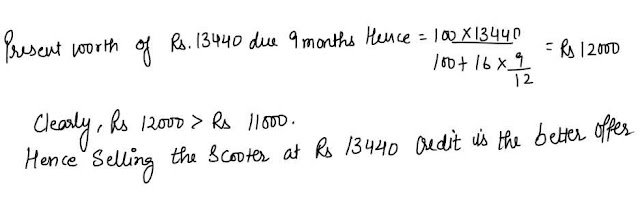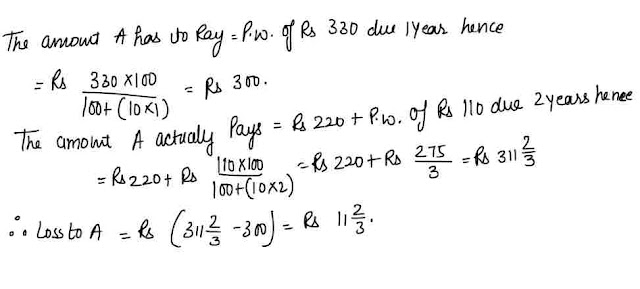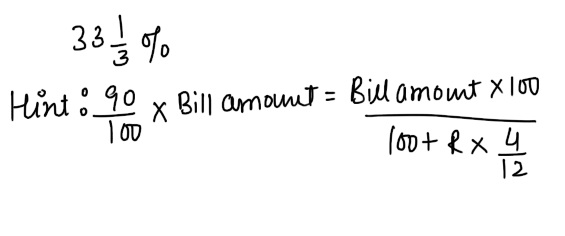# True Discount and Banker's Discount : Concepts with ExamplesPresent Worth : The money to be paid before due date to clear off a debt is called present worth.
True Discount: The difference between the amount and the present worth is called the true discount (T.D.).Also, it is the interest on the present worth (P.W.) for the time which will elapse before the debt is due to be discharged.

#### Formulae#### Banker's Discount:

Let's say that A purchases goods from B.Worth Rs 4000 at a credit of 5 months. B draws up a bill, called the bill of exchange and A signs it thereby allowing B to withdraw the amount from his bank account exactly after 5 months. The bill would appear to be due exactly after 5 months called the normally due date.but in reality it will not be till three days (called as grace days) after the nominally due date. This date is called the legally due date .B can present the bill any day on or legally due date.

Now suppose that B wants to have the money before the legally due date .In this case he sells the bill to a banker or a bill broker.Now the banker would not give the present value of Rs 4000  calculated at simple interest from the day of discounting (i.e.when banker pays the money ) to the o which the bill falls due. For if he does so he would not make any gain. In other words, by deducting the true discount from the face value of the bill he gains nothing.

#### FORMULAE#### Ques 1.

Find the present worth of Rs 1386 due in two equal half-yearly instalments at 10% per annum simple interest.
Ans 1.#### Ques 2.

A merchant owes a businessman Rs 8640 due one year from Now. The merchant wants to settle the accounts after 4 months .If the rate of interest is 12% annum , find the amount he should pay.
Ans 2.#### Ques 3.

Rs 20 is the true discount on Rs 260 due after a certain time.What will be the true discount on the same sum due after a quarter of the former time, the rate of interest being the same ?
Ans 3.#### Ques 4.

A trader buys goods for Rs 400 and sells them the same day for Rs 480 at a credit of 8 months. His gain % is 2% .find the rte of interest .
Ans 4.#### Ques 5.

A man wants to sell his scooter. There are two offers before him __ one at Rs 11000 cash and the other at Rs 13440 credit to be paid after 9 months , the rate of interest being 16% p.a. Which one is the better offer?
Ans 5.#### Ques 6.

A has to pay B Rs 330 after one year .B asks A to pay Rs 220 in cash and after the payment of the rest to the amount for two years and A agrees to it, If the rate of interest be 10% per annum find the loss accured to A.
Ans 6.#### Ques 7.

The banker's discount on Rs 1600 at 6% is the same as the true discount on Rs 1624 for the same time and at the same rate .Find the interval of time between the date of discounting and the legally due date.
Ans 7.#### Ques 8.

A tradesman allows 10% cash discount on a bill which is payable 4 months hence.The rate per cent p.a. customer gets for his money,who pays cash is
(a) 12%
(b) 20%
(c) 33 1/3%
(d) None of these
Ans 8.#### Ques 9.

Banker's gain is 1/10th of true discount for 1 year on a certain sum.The rate per cent p.a. is
(a) 10%
(b) 11 1/9%
(c) 9 1/11%
(d) None of these
Ans 9.#### Ques 10.

Rs 20 is the true discount on Rs 260 due after a certain time. What will be the true discount on the same sum due after half of the former time, the rate of interest being the same?
(a) Rs 10
(b) rs 10.40
(c) Rs 15.20
(d) Rs 13
Ans 10. Rs 10.40

#### Ques 11.

The true discount on a bill due 10 months hence at 6% per annum is Rs 26.25. The amount of the bill is
(a)Rs 1575
(b) Rs 500
(c)Rs 650.25
(d)Rs 551.25
Ans 11. Rs 551.25

#### Ques 12.

If the true discount on a sum due 2years hence at 5% per annum be Rs 75 then the sum due is
(a) Rs 750
(b) Rs 825
(c) Rs 875
(d) Rs 800
Ans 12. Rs 825

#### Ques 13.

True discount on a certain sum of money due 4 years hence is Rs 80 and the simple interest on the same sum for the same time at at the same rate is Rs 100.Determine the Rate %p.a.
(a) 6%
(b) 5%
(c) 7 1/2
(d) 6 1/4%
Ans 13.